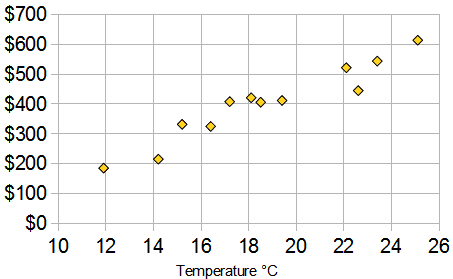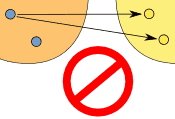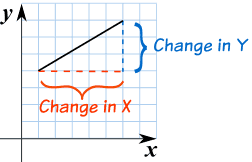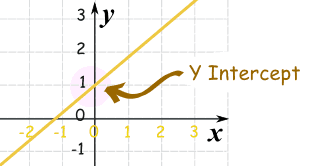Standard

#### The graphs are about having data for two variables (x, y) . Typically the two types of data are related in some way.

Below is a Scatter Plot with bivariate data. The two things that we are comparing are Temperature and Ice Cream Sales.

Here we have ice cream sales versus the temperature on that day. The two variables are Ice Cream Sales and Temperature.

###### (If you have only one set of data, such as just Temperature, it is called “Univariate Data, bivariate mean two types of data)In this scatter plot you can see that as tempurate rises so do prices.

https://www.mathsisfun.com/definitions/bivariate-data.html

Click here and  here   and here and here to practice Identifying Scatter Plot Trends and Predicting with Best Fit Lines online and get automatic feedback (it grades it)! 🙂

## Writing Linear Equations

### Slope Intercept Form:      y = mx + b

The variable m is the slope, it explains the steepness of a line.

The variable b is the y-intercept, this is where the line touches or intersects at the y-axis.

http://www.shmoop.com/video/slope-intercept-form/

Standard

Standard

# Functions

## A Function is Special

A function is a type of equation.

A function has special rules it must follow:

In a function there can ONLY be ONE output(y) for every input (x).

Just like I stated before, a Function is Special! 🙂

• It must work for every possible input value
• And it has only one relationship for each input valueTHIS IS A FUNCTION!                  THIS IS NOT A FUNCTION!

### Example: y = x²

FUNCTIONCould also be written as a table:

X: x Y: x2
3 9
1 1
0 0
4 16
-4 16

It is a function, because:

• Every element in X is related to Y
• No element in X has two or more relationships
• The X values DO NOT repeat

So it follows the rules.

(Notice how both 4 and -4 relate to 16, which is allowed since 4 and -4 are two different numbers.)

### Example: This relationship is not a function:

NOT A FUNCTIONThis is not a function, for these reasons:

• Value “3” in X has no Y
• Value “4” in X has no Y
• Value “5” is related to more than one value in Y
• REMEMBER THE X VALUE CANNOT REPEAT

# Unit Rate

Unit rates are about the amount for 1 unit.

We use Ratio Tables to help solve for rates.
Our Ratio table looks like a tic-tac-toe table.We MUST label our table to know where to put our information

Ex. Bob drives 100 miles in 5 hours. How many miles does he travel every hour?Now we fill in the information that is given.

Bob drives 100 miles in 5 hours. How many miles does he travel every hour?Once we have entered what was given we need to see what the question is asking us.

Ex. Bob drives 100 miles in 5 hours. How many miles does he travel every hour?

Ask yourself, is the problem asking how many hours it takes for 1 mile or how many miles for 1 hour???????

If you said how many miles for 1 hour, you are correct!

So now we enter that into our Ratio Table.The x is the unknown. This is what we are solving for.To solve for x, we multiply 100 and 1. Then divide by the number left over which is 5.

We end up with x = 20.

So Bob drives 20 miles per hour.

Click here to watch a video with a different way to find unit rate.

Test yourself on unit rates here!

# Unit 4: Slope and Y-Interecept

Standard

### Slope Intercept Form:      y = mx + b

The variable m is the slope, it explains the steepness of a line of how slanted the line is.

The variable b is the y-intercept, this is where the line crosses or intersects the y-axis.

https://www.desmos.com/calculator

http://www.shmoop.com/video/slope-intercept-form/

# Slope: m

Slope is how steep a straight line is.
When finding the slope from a line on a graph we use the method of rise over run .
• Rise is how far up
• Run is how far alongYou can also think of it as the change in y over the change in x.EXAMPLE #1:The slope here is 4/6 which can simplify to 2/3.
EXAMPLE #2:In this example the slope is 3/5.

# Practice Problems

TEST YOUR SELF ON LINES HERE!!!!

https://my.hrw.com/wwtb/api/viewer.pl

# Y Intercept: b

Y intercept is where a straight line crosses the Y axis of a graph.

### Example:In the above diagram the line crosses the Y axis at 1.

So the Y intercept is equal to 1.

# Slope Intercept Form

### y = mx + b

m = Slope (how steep the line is)

b = the Y Intercept (where the line crosses the Y axis)\### How do you find “m” and “b”?

• b is easy: just see where the line crosses the Y axis.
• m (the Slope) needs some calculation. Remember to write slope as a fraction.

## Example 1)The fastest and easiest thing to find first when looking at a graph is the y-intercept (b).

Here we see that the line crosses the y-axis at positive 1.

So, b = 1 .

Now to find the slope (m) we will use rise over run:

• Rise is how far up
• Run is how far along

In this example the rise is 2 and the run is 1.

So, m = 2/1 .

Now that I know m = 2/1 and b = 1 I can plug them into the equation for slope intercept form y = mx + b.

y = (2/1) x + 1

^^^^^ This is the equation of the line.